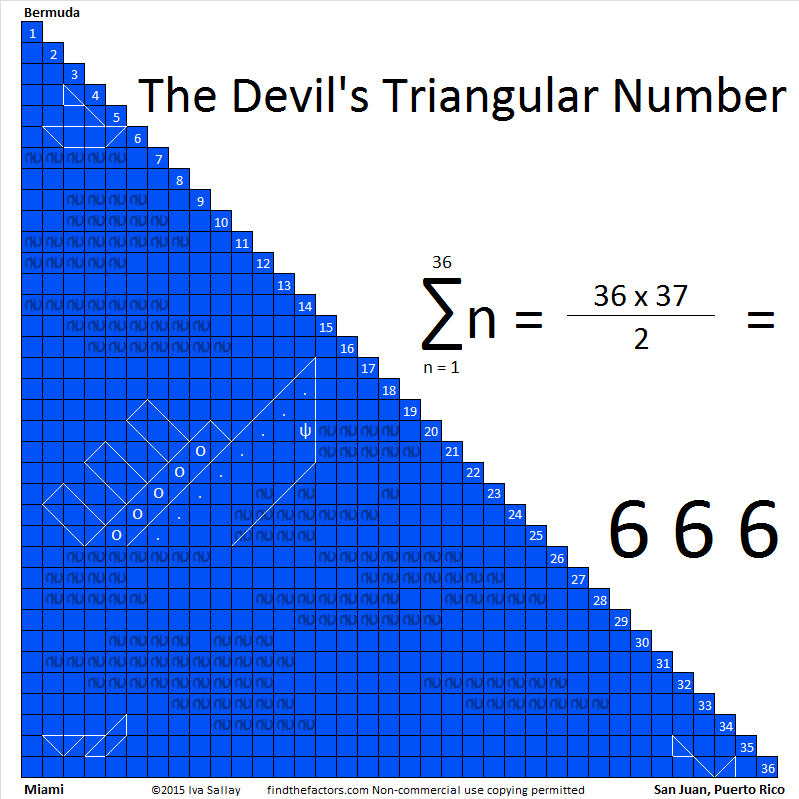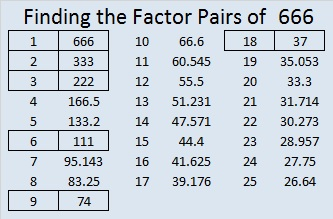# 666 The Devil’s Triangular Number

666 is known as the number of the beast. It is also a triangular number because it is the sum of all the counting numbers from 1 to 36.

I felt a math joke was trying to emerge from those two facts so I made a graphic:The graphic features 666 blue squares and a few sinking boats traveling through the triangular region between Miami, San Juan, and Bermuda. This region is one of the most traveled throughout the world and is also known as the Devil’s triangle because supposedly more ships have disappeared there than in any other similarly sized body of water.

The sum of the numbers on a roulette wheel is also 666. Adding 0 and 00 to the mix does not change that fact. Even though I was born in Las Vegas and lived in one of its suburbs for over 26 years of my life, I have never spun a roulette wheel or gambled in any other way. This number fact is just one more reason not to do it.

Because 37 is one of its factors, 666 is the hypotenuse of the Pythagorean triple 216-630-666. What is the greatest common factor of those three numbers?

Archimedes-lab lists MANY other interesting facts about the number 666. Here are three of my favorites:

• In Roman numerals 600 is DC, 60 is LX, and 6 is VI. Thus DCLXVI is 666 and uses all the Roman numeral characters less than M (1000) exactly one time.
• 15² + 21² = 666; 15 and 21 are both triangular numbers.
• 2² + 3² + 5² + 7² + 11² + 13² + 17² =666; the numbers being squared are the first seven prime numbers.
• 666 is the largest repdigit triangular number. It is also a palindrome.

I saw this next number fact on twitter.

Finally, here is the factoring information for the number 666:

• 666 is a composite number.
• Prime factorization: 666 = 2 x 3 x 3 x 37, which can be written 666 = 2 x (3^2) x 37
• The exponents in the prime factorization are 1, 2, and 1. Adding one to each and multiplying we get (1 + 1)(2 + 1)(1 + 1) = 2 x 3 x 2 = 12. Therefore 666 has exactly 12 factors.
• Factors of 666: 1, 2, 3, 6, 9, 18, 37, 74, 111, 222, 333, 666
• Factor pairs: 666 = 1 x 666, 2 x 333, 3 x 222, 6 x 111, 9 x 74, or 18 x 37
• Taking the factor pair with the largest square number factor, we get √666 = (√9)(√74) = 3√74 ≈ 25.8069758## 8 thoughts on “666 The Devil’s Triangular Number”

1.chrismcmullen

That’s fascinating. I wouldn’t have suspected the mathematics of this one number could seem so rich.

•ivasallay

Yes, this number certainly has a colorful history and some curious properties!

2.abyssbrain

Thanks for linking to my posts 🙂

•ivasallay

You’re welcome. They are very memorable posts.

3.A girl

That means numberblock 666 is a super rectangle and a step squad

•ivasallay

Yes. Thanks for telling me about them. For anyone wanting to know more, here’s an introduction to numberblocks: https://m.youtube.com/watch?v=G5r5-FH7REM

4.Maarten

666 is indeed the 36th triangulair number while 36 is on its turn the 8th triangulair number. In fact 666 is the 8th triangulair – triangulair number in the set [1, 6, 21, 55, 120, 231, 406, 666]. This relation of the number 666 to the number 8 is by some thelogians related to revelations 17: where the beast is identified as the 8th king (and stil one out of 7 kings).

•ivasallay

Wow! Very interesting! Thanks so much for sharing these details.

This site uses Akismet to reduce spam. Learn how your comment data is processed.